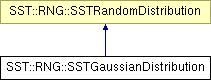# SST::RNG::SSTGaussianDistribution Class Reference

Creates a Gaussian (normal) distribution for which to sample. More...

`#include "sst/core/rng/gaussian.h"`

Inheritance diagram for SST::RNG::SSTGaussianDistribution:## Public Member Functions

SSTGaussianDistribution (double mn, double sd)
Creates a new distribution with a predefined random number generator with a specified mean and standard deviation.
SSTGaussianDistribution (double mn, double sd, SSTRandom *baseRNG)
Creates a new distribution with a predefined random number generator with a specified mean and standard deviation.
~SSTGaussianDistribution ()
Destroys the Gaussian distribution.
double getNextDouble ()
Gets the next double value in the distributon.
double getMean ()
Gets the mean of the distribution.
double getStandardDev ()
Gets the standard deviation of the distribution.

## Protected Attributes

double mean
The mean of the Gaussian distribution.
double stddev
The standard deviation of the Gaussian distribution.
SSTRandombaseDistrib
The base random number generator for the distribution.
double unusedPair
Random numbers for the distribution are read in pairs, this stores the second of the pair.
bool usePair
Random numbers for the distribution are read in pairs, this tells the code to use the second of the pair.
bool deleteDistrib
Controls whether the destructor deletes the distribution (we need to ensure we do this IF we created the distribution).

## Detailed Description

Creates a Gaussian (normal) distribution for which to sample.

## Constructor & Destructor Documentation

 SST::RNG::SSTGaussianDistribution::SSTGaussianDistribution ( double mn, double sd ) ` [inline]`

Creates a new distribution with a predefined random number generator with a specified mean and standard deviation.

Parameters:
 mn The mean of the Gaussian distribution sd The standard deviation of the Gaussian distribution

References baseDistrib, deleteDistrib, mean, stddev, unusedPair, and usePair.

 SST::RNG::SSTGaussianDistribution::SSTGaussianDistribution ( double mn, double sd, SSTRandom * baseRNG ) ` [inline]`

Creates a new distribution with a predefined random number generator with a specified mean and standard deviation.

Parameters:
 mn The mean of the Gaussian distribution sd The standard deviation of the Gaussian distribution baseRNG The random number generator as the base of the distribution

References baseDistrib, deleteDistrib, mean, stddev, unusedPair, and usePair.

## Member Function Documentation

 double SST::RNG::SSTGaussianDistribution::getMean ( ) ` [inline]`

Gets the mean of the distribution.

Returns:
The mean of the Guassian distribution

References mean.

 double SST::RNG::SSTGaussianDistribution::getNextDouble ( ) ` [inline, virtual]`

Gets the next double value in the distributon.

Returns:
The next double value of the distribution (in this case a Gaussian distribution)

Implements SST::RNG::SSTRandomDistribution.

References baseDistrib, mean, SST::RNG::SSTRandom::nextUniform(), stddev, unusedPair, and usePair.

 double SST::RNG::SSTGaussianDistribution::getStandardDev ( ) ` [inline]`

Gets the standard deviation of the distribution.

Returns:
The standard deviation of the Gaussian distribution

References stddev.

The documentation for this class was generated from the following file:

Generated on 14 Sep 2015 for SST by1.6.1